# Match Topology

Fix gaps between stitched edges and vertices to make the actual geometry of the surfaces consistent with the model topology.

1. From the menu bar, click Geometry > Geom Match Topology.
The Geom Match Topology dialog opens.
2. Using the Entities selector, select geometry to check.
3. In the Tolerance field, enter a tolerance to use when checking the topology of the model for gaps between shared, non-manifold, or suppressed edges.

If a gap is found to be bigger than the specified tolerance, then the surface and edge geometries will be morphed parametrically and in 3D, if necessary, to make the gap smaller than the tolerance. In addition, non-essential degenerate edges are removed.

4. Click Apply.

The original geometric entity IDs are also preserved. The same functionality is used for the Optimize for CAD option in the Export - Geometry Browser.

## Example: Match Topology

This example demonstrates the difference in results when using the Geom Match Topology tool to update geometry that has previously been repaired with topology-based geometry cleanup operations, for example, toggle/equivalence/replace edges, replace points, and so on. The geometry below was repaired using only topology-based geometry cleanup operations. After the geometry was meshed, you can see that some of the elements have become distorted. After untoggling the edges, there is still a large gap in the geometry.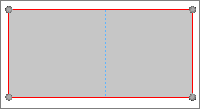Figure 2. Repaired Geometry not Updated with the Geom Match Topology Tool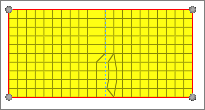Figure 3. Meshed Geometry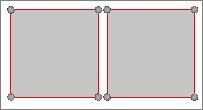Figure 4. Geometry with Edges Untoggled
The geometry below was repaired using topology-based geometry cleanup operations and then updated with the Geom Match Topology tool. After the geometry was meshed, you can see that there are no distorted elements. After untoggling the edges, there is not a gap in the geometry.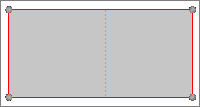Figure 5. Repaired Geometry Updated with the Geom Match Topology Tool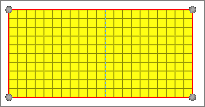Figure 6. Meshed Geometry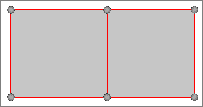Figure 7. Geometry with Edges Untoggled